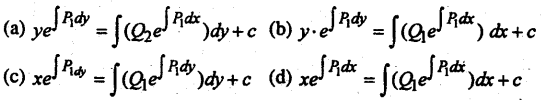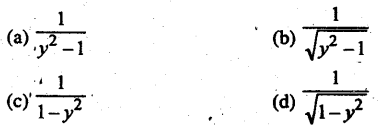# Bihar Board 12th Maths Objective Important Questions Part 4

## Bihar Board 12th Maths Objective Important Questions Part 4Question 1.
The order of the differential equation $$2 x^{2} \frac{d^{2} y}{d x^{2}}+3 \frac{d y}{d x}+y=0$$ is ’
(a) 3
(b) 2
(c) 1
(d) not defined
(a) 3

Question 2.
The number of arbitrary constants in the general solution of a differential equation of fourth order are.
(a) 0
(b) 2
(c) 3
(d) 4
(d) 4

Question 3.
The number of arbitrary constants in the particular solution of a differential equation of third order are :
(a) 3
(b) 2
(c) 1
(d) 0
(d) 0

Question 4.
Which of the following differential equations hasy = c1ex + c2e-x as the general solution?
(a) $$\frac{d^{2} y}{d x^{2}}$$ + y = 0
(b) $$\frac{d^{2} y}{d x^{2}}$$ – y = 0
(c) $$\frac{d^{2} y}{d x^{2}}$$ + 1 = 0
(d) $$\frac{d^{2} y}{d x^{2}}$$ – 1 = 0
(b) $$\frac{d^{2} y}{d x^{2}}$$ – y = 0Question 5.
Which of the following differential equations has y =x as one crits particular solution ?(c)

Our free handy Double Integral Calculator tool is aimed at giving the double integral of a function within fraction of seconds.

Question 6.
The general solution of the differential equation $$\frac{d y}{d x}$$ = ex+y is ;
(a) ex + e-xy = c
(b) ex + ey = c
(c) e-x + ey = c
(d) e-x + e-y = c
(a) ex + e-xy = c

Question 7.
A homogeneous differential equation of the front $$\frac{d x}{d y}=h\left(\frac{x}{y}\right)$$ can be solved by marking the substitution : .
(a) y = Vx
(b) V = yx
(c) x = Vy
(d) x = V
(c) x = Vy

Question 8.
Which of the following is a homogeneous differential equation :
(a) (4x + 6y + 5) dy – |3y + 2x + 4| dx = 0
(b) (xy) dx – (x3 + y3) dy = 0
(c) (x3 + 2y2)dx + 2xydy = 0
(d) y2dx + (x2 – xy – y3)dy = 0
(d) y2dx + (x2 – xy – y3)dy = 0Question 9.
The integrating factor of the differential equation $$x \frac{d y}{d x}-y=2 x^{2}$$ is :
(a) e-x
(b) e-y
(c) 1/x
(d) x
(c) 1/x

Question 10.
The general solution of a differential equation of the type $$\frac{d x}{d y}+P_{1} x=Q_{1}$$ is :(c)

Question 11.
The integrating factor of the differential equation
(a-y2)$$\frac{d x}{d y}$$(d) $$\frac{1}{\sqrt{1-y^{2}}}$$

Question 12.
In triangle ABC, which of the following is not true :(c)

Question 13.
If $$\vec{a}$$ and $$\vec{b}$$ are two colliner vectors, then which of the following are incorrect:
(a) $$\vec{b}$$ = λ $$\vec{a}$$, for some scalar λ
(b) $$\vec{a}$$ = ±$$\vec{b}$$
(c) The respective compounds of $$\vec{a}$$ and $$\vec{b}$$ are proportional
(d) Both the vectors $$\vec{a}$$ and $$\vec{b}$$ have some direction, but different magnitudes.
(d) Both the vectors $$\vec{a}$$ and $$\vec{b}$$ have some direction, but different magnitudes.

Question 14.
Let the vectors $$\vec{a}$$ and $$\vec{b}$$ be such that |$$\vec{a}$$| = 3 and |$$\vec{a}$$|= $$\frac{\sqrt{2}}{3}$$, then $$\overrightarrow{a} \times \overrightarrow{b}$$ is a unit vector, If the angle between $$\vec{a}$$ and $$\vec{b}$$ is :
(a) $$\frac{\pi}{6}$$
(b) $$\frac{\pi}{4}$$
(c) $$\frac{\pi}{3}$$
(d) $$\frac{\pi}{2}$$
(b) $$\frac{\pi}{4}$$Question 15.
Area of a rectangle having vertics A, B, C and D with position :
Vectors $$-\hat{i}+\frac{1}{2} \hat{j}+4 \hat{k}, \hat{i}+\frac{1}{2} \hat{j}+4 \hat{k}, \hat{i}-\frac{1}{2} \hat{j}+4 \hat{k}$$ and $$-\hat{i}-\frac{1}{2} \hat{j}+4 \hat{k}$$ respectively is:
(a) $$\frac { 1 }{ 2 }$$
(b) 1
(c) 2
(d) 4
(c) 2

Question 16.
If $$\vec{a}$$ is a nonzero vector of magnitude ‘a’ and λ nonzero scalar, then λ$$\vec{a}$$ is unit vector If,
(a) λ = 1
(b) λ = -1
(c) a = |λ|
(d) a = $$\frac{1}{|\lambda|}$$
(d) a = $$\frac{1}{|\lambda|}$$

Question 17.
The general solution of the differential equation
exdy + (yex +2x)dx = 0 is :
(a) xex + x2 = c
(b) xey + y2 = c
(c) yex + x2 = c
(d) yey + x2 = c
(c) yex + x2 = c

Question 18.
If θ is the angle between two vectors $$\vec{a}$$ and $$\vec{b}$$, the $$\vec{b}$$, $$\vec{a}$$, $$\vec{b}$$ ≥ 0 only when :
(a) 0 < θ < $$\frac{\pi}{2}$$
(b) 0 ≤ θ ≤ $$\frac{\pi}{2}$$
(c) 0 < θ < π
(d) 0 ≤ θ ≤ π
(b) 0 ≤ θ ≤ $$\frac{\pi}{2}$$Question 19.
Let $$\vec{a}$$ and $$\vec{b}$$ be two unit vectors and θ is the angle between them; Then $$\vec{a}$$ + $$\vec{b}$$ is a unit vector if:
(a) θ = $$\frac{\pi}{4}$$
(b) θ = $$\frac{\pi}{3}$$
(c) θ = $$\frac{\pi}{2}$$
(d) θ = $$\frac{2\pi}{3}$$
(d) θ = $$\frac{2\pi}{3}$$

Question 20.
The value of $$\hat{i}(\hat{j} \times \hat{k})+\hat{j}(\hat{i}+\hat{k})+\hat{k}(\hat{i}+\hat{j})$$ is :
(a) 0
(b) -1
(c) 1
(d) 3
(c) 1

Question 21.
If θ is the angle between any two vectors $$\vec{a}$$ and $$\vec{b}$$ the $$|\vec{a} \cdot \vec{b}| \vec{a} \cdot \vec{b}|=| \vec{a} \times \vec{b} \mid$$ when θ, is equal to
(a) 0
(b) $$\frac{\pi}{4}$$
(c) $$\frac{\pi}{2}$$
(d) π
(b) $$\frac{\pi}{4}$$

Question 22.
The planes 2x – y + 4z = 5 and Sx – 2.5y + 10z = 6 are :
(a) perpendicular
(b) parallel
(c) intersect y-axis
(d) passes through (0, 0, $$\frac{5}{4}$$
(b) parallel

Question 23.
Distance between the two planes 2x + 3 y +-4z = 4 and 4x + 6y + 8x = 12 is
(a) 2 units
(b) 4 units
(c) 8 units
(d) 9 units
(d) 9 units

Question 24.
The solution set of the in equation 2x + y > 5 is :
(a) half plane that contains the origin
(b) open half plane not containing the origin
(c) when xy-plane except the reints lying on the live 2x + y = 5
(d) none of these
(b) open half plane not containing the origin

Question 25.
Which of the following is not a convex set ?
(a) {(x, y)/2x + 5y < 7}
(b) {(x,y)/x2 + y2 ≤ 4} .
(c) {x/ |x| = 5}
(d) {(x,y)/3x2 + 2y ≤ b
(c) {x/ |x| = 5}

Question 26.
The reints of which the maximum value of x + y. Subject to the constraints x + 2y ≤ 70, 2x + y ≤ 95,x,y ≥ 0 is obtained is :
(a) (30,25)
(b) (20,35)
(c) (35,20)
(d) (40,15)
(d) (40,15)Question 27.
Objective function of a LPP is :
(a) a constraint
(b) a function to be optimized
(c) a relation b/w the variables
(d) none of these
(b) a function to be optimized

Question 28.
The Probability of obtaining an even prime number on each die, when a pair of dice is rolled is :
(a) 0
(b) $$\frac { 1 }{ 3 }$$
(c) $$\frac { 1 }{ 12 }$$
(d) $$\frac { 1 }{ 36 }$$
(d) $$\frac { 1 }{ 36 }$$

Question 29.
If P (A) = $$\frac { 1 }{ 2 }$$ P(B) = 0 then P(A/B) is :
(a) 0
(b) $$\frac { 1 }{ 2 }$$
(c) not defined
(d) 1
(c) not defined

Question 30.
If Aand B are two events such that P(A) ≠ 0 and P(B/A) = then :
(a ) A⊂B
(b ) B⊂A
(c) B = Φ
(d) A = Φ
(a ) A⊂B

Question 31.
If P(B/A)>P(A) then which of the following is correct:
(a) P(B / A) < P(B)
(b) P(AnB)<P(A) P(B) (c) P(B/ A) > P(B)
(d) P(B/A) = P(B)
(c) P(B/ A) > P(B)Question 32.
Two events A and B will be independent, if:
(a) A and B are mutually exclusive
(b) P(A’B’)=[1-P(A)][1-P(B)]
(c )P(A) = P(B)
(d) P(A) + P(B) = 1
(b) P(A’B’)=[1-P(A)][1-P(B)]

Question 33.
If A and B are any two events such that P(A) + P(B) – P (A and B) = P(A), the ;
(a) P(B/A) = 1
(b) P(A/B) = 1
(c) P(B/A) = 0
(d) P(A/B) = 0
(b) P(A/B) = 1

Question 34.
Suppose that two cards are drown at random from a deck of cards. Let X be the number of aces obtained. Then the. value of E(X) is :
(a) $$\frac{37}{221}$$
(b) $$\frac{9}{13}$$
(c) $$\frac{1}{13}$$
(d) $$\frac{2}{13}$$
(d) $$\frac{2}{13}$$

Question 35.
In a box containing 100 bulbs. 10 are defective. The probability that out of a sample of 5 bulbs, none in defective is :
(a) 10-1
(b) $$\left(\frac{1}{2}\right)^{5}$$
(c) $$\left(\frac{9}{10}\right)^{5}$$
(d) $$\frac{9}{10}$$
(c) $$\left(\frac{9}{10}\right)^{5}$$

Question 36.
The probability that a student Is not a swimmer is $$\frac{1}{5}$$ then the probability that mat of five students four are swimmers is:
(a) $${ }^{5} C_{4}\left(\frac{4}{5}\right)^{4} \frac{1}{5}$$
(b) $$\left(\frac{4}{5}\right)^{4} \frac{1}{5}$$
(c) $${ }^{5} C_{1} \frac{1}{5}\left(\frac{4}{5}\right)^{4}$$
(d) None of these
(a) $${ }^{5} C_{4}\left(\frac{4}{5}\right)^{4} \frac{1}{5}$$

Question 37.
The mean of the numbers of obtained on throwing a die having written. I on three facems, 2 on two faces and 5 on one face is :
(a) 1
(b) 2
(c) 5
(d) $$\frac { 8 }{ 3 }$$
(b) 2Question 38.
If y = log x then $$\frac{d^{2} y}{d x^{2}}$$ =
(a) log x
(b) $$\frac{1}{x}$$
(c) $$-\frac{1}{x^{2}}$$
(d) $$\frac{1}{x^{2}}$$
(c) $$-\frac{1}{x^{2}}$$

Question 39.
$$\frac{d}{d x}\left[\frac{d}{d x}(\sin x)\right]=$$
(a) sin x
(b) cos x
(c) -sin x
(d) -cos x
(c) -sin x

Question 40.
$$\int_{a}^{b}\left[\frac{d}{d x}(\log x)\right]=$$
(a) log $$\frac{b}{a}$$
(b) log $$\frac{a}{b}$$
(c) log(a-b)
(d) log(b-a)
(b) log $$\frac{a}{b}$$

Question 41.
dy – dx = y – x hindi
(a) y + x = k
(b) y – x = k
(c) y/x = k
(d) xy = k
(b) y – x = k

Question 42.
$$|2 \vec{i}+3 \vec{j}+5 \vec{k}|$$
(a) 10
(b) $$\sqrt{10}$$
(c) 38
(d) $$\sqrt{38}$$
(d) 0Question 43.
$$(5 \vec{i}+2 \vec{j}-\vec{k}) \cdot(\vec{i}-2 \vec{j}-\vec{k})=$$
(a) 13
(b) 8
(c) 6
(d) 0
(d) 0

Question 44.
$$\frac{d}{d x}$$ [(sin-1x + cos-1x)] =
(a) $$\frac{\pi}{2}$$
(b) 0
(c) $$\frac{\pi^{2}}{2}$$
(d) 1